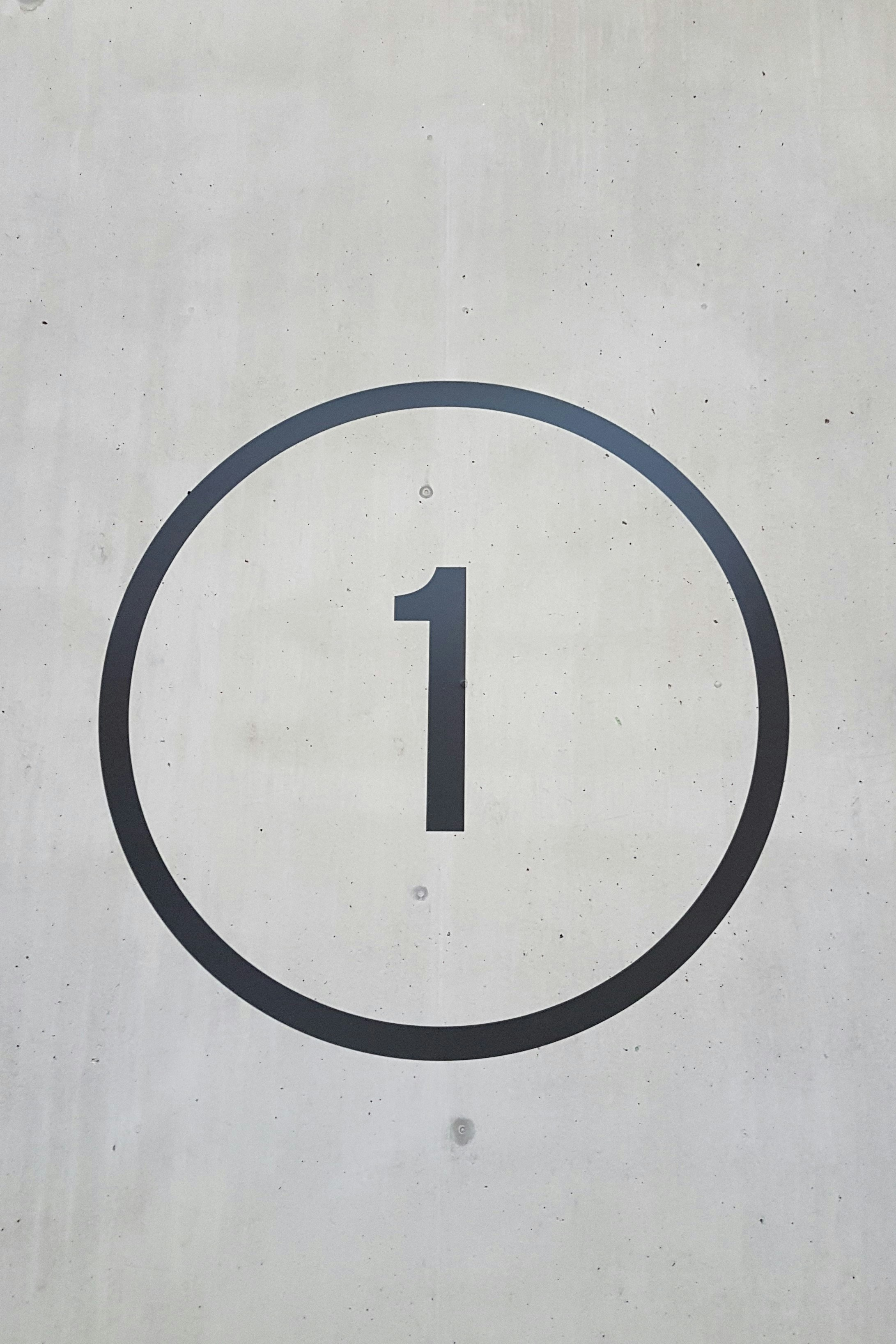Hackerrank - Modified Kaprekar Numbers Solution

# Hackerrank - Modified Kaprekar Numbers SolutionA modified Kaprekar number is a positive whole number with a special property. If you square it, then split the number into two integers and sum those integers, you have the same value you started with.

Consider a positive whole number  with  digits. We square  to arrive at a number that is either  digits long or  digits long. Split the string representation of the square into two parts,  and . The right hand part,  must be  digits long. The left is the remaining substring. Convert those two substrings back to integers, add them and see if you get .

For example, if ,  then . We split that into two strings and convert them back to integers  and . We test , so this is not a modified Kaprekar number. If , still , and . This gives us , the original .

Note: r may have leading zeros.

Here's an explanation from Wikipedia about the ORIGINAL Kaprekar Number (spot the difference!):

In mathematics, a Kaprekar number for a given base is a non-negative integer, the representation of whose square in that base can be split into two parts that add up to the original number again. For instance, 45 is a Kaprekar number, because 45² = 2025 and 20+25 = 45.

Given two positive integers  and  where  is lower than , write a program to print the modified Kaprekar numbers in the range between  and , inclusive.

Function Description

Complete the kaprekarNumbers function in the editor below. It should print the list of modified Kaprekar numbers in ascending order.

kaprekarNumbers has the following parameter(s):

• p: an integer
• q: an integer

Input Format

The first line contains the lower integer limit .
The second line contains the upper integer limit .

Note: Your range should be inclusive of the limits.

Constraints

Output Format

Output each modified Kaprekar number in the given range, space-separated on a single line. If no modified Kaprekar numbers exist in the given range, print INVALID RANGE.

Sample Input

1
100


Sample Output

1 9 45 55 99

Explanation

, , , , and  are the Kaprekar Numbers in the given range.

### Solution in Python

def check(i):
sq = str(i**2)
le = len(str(i))
r = sq[-le:]
l = sq[:-le] or '0'
return sum(map(int,(l,r)))==i

def kaprekarNumbers(p, q):
return [i for i in range(p,q+1) if check(i)]

p = int(input())
q = int(input())
print(*kaprekarNumbers(p, q) or ["INVALID RANGE"])